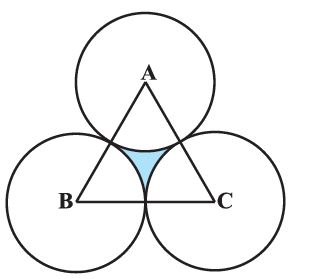In the verge of coronavirus pandemic, we are providing FREE access to our entire Online Curriculum to ensure Learning Doesn't STOP!

# Ex.12.3 Q10 Areas Related to Circles Solution - NCERT Maths Class 10

Go back to  'Ex.12.3'

## Question

The area of an equilateral triangle $$ABC$$ is $$17320.5\, \rm{cm}^2.$$ With each vertex of the triangle as center, a circle is drawn with radius equal to half the length of the side of the triangle (see Figure). Find the area of the shaded region.

(Use $$\pi = 3.14$$ and $$\sqrt 3 = 1.73205$$)Video Solution
Areas Related To Circles
Ex 12.3 | Question 10

## Text Solution

What is known?

(i) Area of an equilateral triangle $$\Delta {ABC} = 17320.5\,{\text{c}}{{\text{m}}^2}$$

(ii) with each vertex of a $$\Delta$$ as center a circle is drawn with radius

\begin{align} = \frac{1}{2}({\text{ length of side of }}\Delta {ABC})\end{align}

(Use $$\pi = 3.14$$ and $$\sqrt 3 = 1.73205$$)

What is unknown?

Reasoning:

(i) Since area of triangle is given , we can find side of $$\Delta {ABC}$$ using the formula of area of equilateral

\begin{align}\Delta = \frac{{\sqrt 3 }}{4}{({\text{side}})^2}\\{({\text{side}})^2} = \frac{{{\text{area}} \times 4}}{{\sqrt 3 }}\end{align}

(ii) Since radius\begin{align} {(r)} = \frac{1}{2} \end{align}( length of side of $$\Delta$$ ) we can find  $$\rm{}r$$

Also, all angles of an equilateral triangle are equal.

$$\therefore \;$$ Angle subtended by each sector \begin{align}(\theta ) = \frac{{{{180}^0}}}{3} = {60^\circ }\end{align}

Using the formula

Area of the sector of angle \begin{align} \theta = \frac{\theta }{{{{360}^ \circ }}} \times \pi {r^2}\end{align}

We can find Area of each sector \begin{align} = \frac{{60}}{{360}} \times \pi {r^2}\, = \frac{\pi }{6}{r^2}\end{align}

All sectors are equal as they have same radius $${r}$$ and \begin{align}{ \theta = 6}{{\text{0}}^{{o}}}\end{align}

(iii) Visually from the figure it is clear that:

Area of the shaded region $$=$$  Area of $$\Delta {ABC} - 3\; \times$$ Area of each sector

\begin{align}&= 17320.5 - 3 \times \frac{{\pi {r^2}}}{6}\\ &= 17320.5 - \frac{{\pi {r^2}}}{2}\end{align}

which can be easily solved using $$\pi = 3.14$$ (as given) and we already know $$\rm{}r$$

Steps:

(i) Area of equilateral $$\Delta = 17320.5\,{\text{c}}{{\text{m}}^2}$$

\begin{align}\frac{{\sqrt 3 }}{4}{({\text{side}})^2} &= 17320.5\,\,{\text{c}}{{\text{m}}^2}\\{\left( {{\text{side}}} \right)^2} &= \frac{{17320.5 \times 4}}{{\sqrt 3 }}\\&= { \frac{{17320.5 \times 4}}{{1.73205}}}\\&{\rm side = \sqrt {10000 \times 4} }\\&= { 100 \times 2}\\& ={ 200\,\,{\text{cm}}}\end{align}

$$\text{radius of each sector(r)}$$\begin{align}&= \frac{1}{2}\left( \rm{side} \right) \\&= \frac{1}{2}\left( {200} \right)\,{\text{cm}}\\ &= 100\,{\text{cm}}\end{align}

All interior angles of an equilateral $$\Delta$$ are of measure $${60^ \circ }$$

And all $$3$$ sectors are made using these interior angles.

$$\therefore\;$$ Angles subtended at the center by each sector $$(\theta) = {60^ \circ }$$

\begin{align}\text{Area of each sector}= \frac{{\rm{\theta }}}{{{{360}^ \circ }}} \times \pi {r^2}\end{align}

$$\text{Area of 3 sectors}$$\begin{align} &= 3 \times \frac{{{{60}^ \circ }}}{{{{360}^ \circ }}} \times \pi {r^2}\\&= 3 \times \frac{1}{6} \times 3.14 \times {\left( {100\rm{}\,cm} \right)^2}\\&= 15700\rm{}\,cm^2 \end{align}

Area of shaded region $$=$$ Area of Quadrant $$\rm{}\Delta{ABC}\,-$$ Area of 3 sector

\begin{align}&= 17320.5c{m^2} -15700c{m^2}\\&= 1620.5c{m^2}\end{align}

Learn from the best math teachers and top your exams

• Live one on one classroom and doubt clearing
• Practice worksheets in and after class for conceptual clarity
• Personalized curriculum to keep up with school